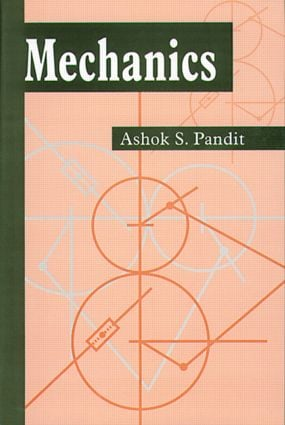# Mechanics

## 1st Edition

Narosa

190 pages

##### Purchasing Options:\$ = USD
Hardback: 9780849309793
pub: 2000-11-13
\$60.95
x

FREE Standard Shipping!

### Description

Based on a course developed by the author, Mechanics provides an introduction to classical mechanics. Each chapter begins with an introduction to the topics under discussion and is followed by precise definitions, propositions, and theorems and ends with exercises that reinforce students understanding. The treatment of the subject is mathematical, but the mathematics used is elementary. In fact, all the mathematics presented involve basic vector algebra and differential calculus of vector valued functions of a real variable. The author avoids explaining the underlying geometry in an explicit manner. Instead, he presents geometric discussions in a kinematical disguise - a treatment beneficial not only to the mathematics student but also to students of physics and engineering.

Preface

BASIC CONCEPTS OF MOTION

Introduction

Concepts of a Particle

Motion of a Particle

Motion of a Plane

Polar Coordinates

Equation of Motion

RECTILINEAR MOTION

Introduction

Equation of Rectilinear Motion

Integration of Equation of Motion

Some Qualitative Analysis

FIRST INTEGRALS OF MOTION

Introduction

Conservative Force Fields

Energy, Momentum and Torque

Conservation Principles

Use of Conservation Principles

CENTRAL FORCE FIELDS

Introduction

Basic Properties

Equation of Motion in Polar Coordinates

Integration of Equations of Motion

An Explanation

Circular Orbits

Force Field from Equation of Orbit

Planetary Motion (Kebler's Problem)

SYSTEM OF PARTICLES

Introduction

A System of Particles

The Center of Mass

Some More Definitions

Conservation Principles

The Two Body Problem

Some Solved Examples

RIGID DYNAMICS

Introduction

The Nature of Motion

The Instantaneous Angular Velocity

Equations of Motion

The Inertia Tensor

Euler's Equations

Symmetric Rigid Bodies

LAGRANGIAN MECHANICS

Introduction

Generalized Coordinates

Motion

The Lagrangian Function

Lagrange's Equations

Hamilton's Principle of Least Action

Hamilton's Equations of Motion

Conservation Principles